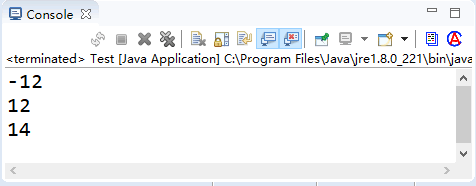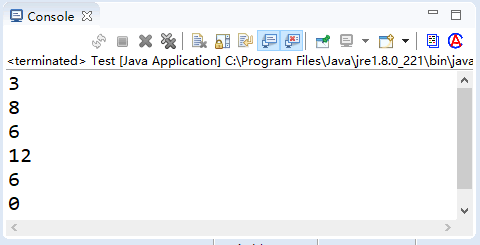# Java算术运算符

Java 语言中的运算符除了具有优先级之外，还有一个结合性的特点。当一个表达式中出现多种运算符时，执行的先后顺序不仅要遵守运算符优先级别的规定，还要受运算符结合性的约束，以便确定是自左向右进行运算还是自右向左进行运算。这些运算符按照操作数的数量可以分为单目运算符、双目运算符和三目运算符。

Java 中的算术运算符主要用来组织数值类型数据的算术运算，按照参加运算的操作数的不同可以分为一元运算符和二元运算符。

## 一元运算符

++ 自加一 先取值再加一，或先加一再取值 a++ 或 ++a
-- 自减一 先取值再减一，或先减一再取值 a-- 或 --a

```int a = 12;
System.out.println(-a);
int b = a++;
System.out.println(b);
b = ++a;
System.out.println(b);```## 二元运算符

Java 语言中算术运算符的功能是进行算术运算，除了经常使用的加（+）、减（-）、乘（*）和除（\）外，还有取模运算（％）。加（+）、减（-）、乘（*）、除（\）和我们平常接触的数学运算具有相同的含义。具体说明参见表 2。

+ 求 a 加 b 的和，还可用于 String 类型，进行字符串连接操作 a + b
- 求 a 减 b 的差 a - b
* 求 a 乘以 b 的积 a * b

%

```int a = 4, b = 2, c = 3;
int d = a * (b + c) % c;```

①int x=2,y=1; 表达式 y/x 的结果是 0。
②float x=2.0f; int y=1; 表达式 y/x 的结果是 0.5。

【例1】编写一个程序，输出不同类型的两个数，执行相加、相减、相乘、相除和求余后输入结果。

```public static void main(String[] args) {
float f1 = 9 % 4;// 保存取余后浮点类型的结果
double da = 9 + 4.5; // 双精度加法
double db = 9 - 3.0; // 双精度减法
double dc = 9 * 2.5; // 双精度乘法
double dd = 9 / 3.0; // 双精度除法
double de = 9 % 4; // 双精度取余

System.out.println("整数的算术运算"); // 整数的加、减、乘、除和取余
System.out.printf("9+4=%d \n", 9 + 4);
System.out.printf("9-4=%d \n", 9 - 4);
System.out.printf("9*4=%d \n", 9 * 4);
System.out.printf("9/4=%d \n", 9 / 4);
System.out.printf("9%%4=%d \n", 9 % 4);

System.out.println("\n浮点数的算术运算"); // 浮点数的加、减、乘、除和取余
System.out.printf("9+4.5f=%f \n", 9 + 4.5f);
System.out.printf("9-3.0f=%f \n", 9 - 3.0f);
System.out.printf("9*2.5f=%f \n", 9 * 2.5f);
System.out.printf("9/3.0f=%f \n", 9 / 3.0f);
System.out.printf("9%%4=%f \n", f1);

System.out.println("\n双精度数的算术运算"); // 双精度数的加、减、乘、除和取余
System.out.printf("9+4.5=%4.16f \n", da);
System.out.printf("9-3.0=%4.16f \n", db);
System.out.printf("9*2.5=%4.16f \n", dc);
System.out.printf("9/3.0=%4.16f \n", dd);
System.out.printf("9%%4=%4.16f \n", de);

System.out.println("\n字符的算术运算"); // 对字符的加法和减法
System.out.printf("'A'+32=%d \n", 'A' + 32);
System.out.printf("'A'+32=%c \n", 'A' + 32);
System.out.printf("'a'-'B'=%d \n", 'a' - 'B');
}```

```整数的算术运算
9+4=13
9-4=5
9*4=36
9/4=2
9%4=1

9+4.5f=13.500000
9-3.0f=6.000000
9*2.5f=22.500000
9/3.0f=3.000000
9%4=1.000000

9+4.5=13.5000000000000000
9-3.0=6.0000000000000000
9*2.5=22.5000000000000000
9/3.0=3.0000000000000000
9%4=1.0000000000000000

'A'+32=97
'A'+32=a
'a'-'B'=31```

## 算术赋值运算符

+= 加赋值 a += b、a += b+3
-= 减赋值 a -= b
*= 乘赋值 a *= b
/= 除赋值 a /= b
%= 取余赋值 a %= b

```int a = 1;
int b = 2;
a += b; // 相当于 a = a + b
System.out.println(a);
a += b + 3; // 相当于 a = a + b + 3
System.out.println(a);
a -= b; // 相当于 a = a - b
System.out.println(a);
a *= b; // 相当于 a=a*b
System.out.println(a);
a /= b; // 相当于 a=a/b
System.out.println(a);
a %= b; // 相当于 a=a%b
System.out.println(a);```